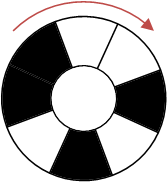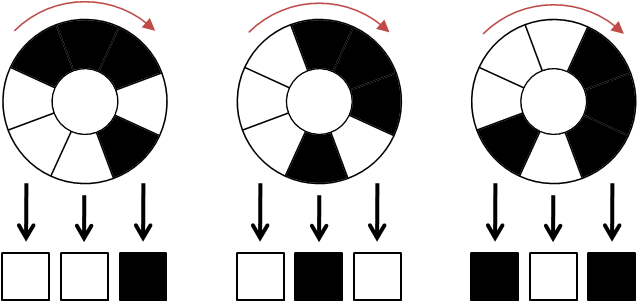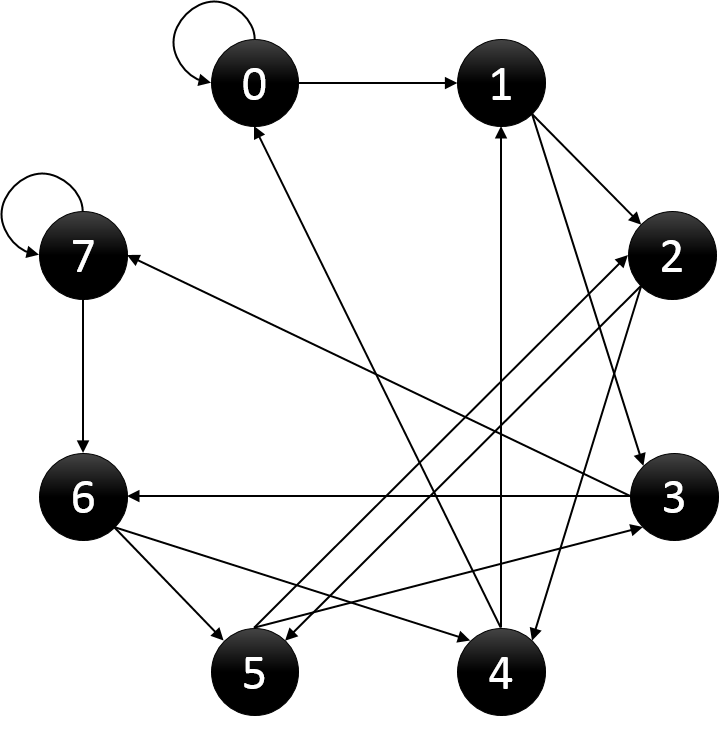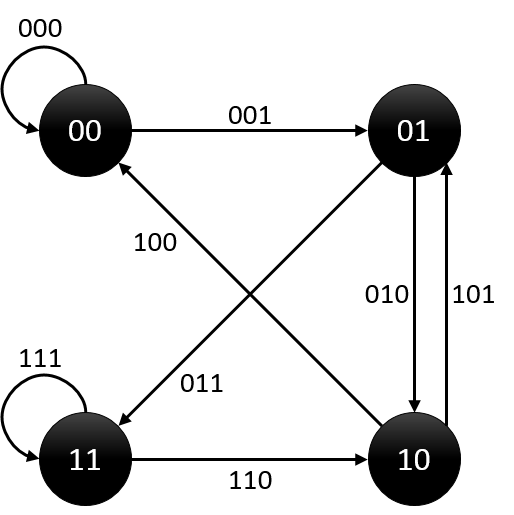# Hihocoder1182 欧拉路·三（欧拉回路+构造）

## 欧拉路·三

• 时间限制:10000ms
• 单点时限:1000ms
• 内存限制:256MB

### 描述机关黑色的部分表示为1，白色的部分表示为0，逆时针连续N个区域表示一个二进制数。打开机关的条件是合理调整圆环黑白两种颜色的分布，使得机关能够表示0~2^N-1所有的数字。

——By 无名的冒险者### 样例输入

3

### 样例输出

00010111

### 链接

http://hihocoder.com/problemset/problem/1182

### 代码

StatusAccepted
Time21ms
Memory5120kB
Length796
#include <iostream>
#include <algorithm>
#include <cstdio>
#include <cstring>
#include <vector>
using namespace std;
const int maxn = 400010;
int n;
int G[maxn];
vector <int> V;

void init()
{
memset(G, -1, sizeof(G));
V.clear();
}

int cnt = 0;

void Euler(int u) // 欧拉回路
{
for (int i = 0; i < 2; ++i)
{
int v = G[u][i];
if (G[u][i] > -1)
{
G[u][i] = -1;
Euler(v);
}
}
V.push_back((u&1)); // 保存最后一位
}

int main()
{
int u;
scanf("%d", &u);
init();
n = (1 << (u -1)); // 点的数量
for (int i = 0; i < n; ++i)
{
int j = ((i << 1) & (n - 1)); // 建图
G[i] = j; // 每个点最多能连两条边，0或1
G[i] = j + 1;
}
Euler(0);
for (int i = V.size() - 1; i > 0; --i) // 倒序输出，最后一位顶点起点重合
printf("%d", V[i]);
printf("\n");
return 0;
}

The end.
2018-07-27 星期五

### 提示：有向图欧拉回路

< 十分钟后 >DFS(u):
While (以u为起点，且未被删除的边e(u,v))
删除边e(u,v)
DFS(v)
End
PathSize ← PathSize + 1
Path[ PathSize ] ← u